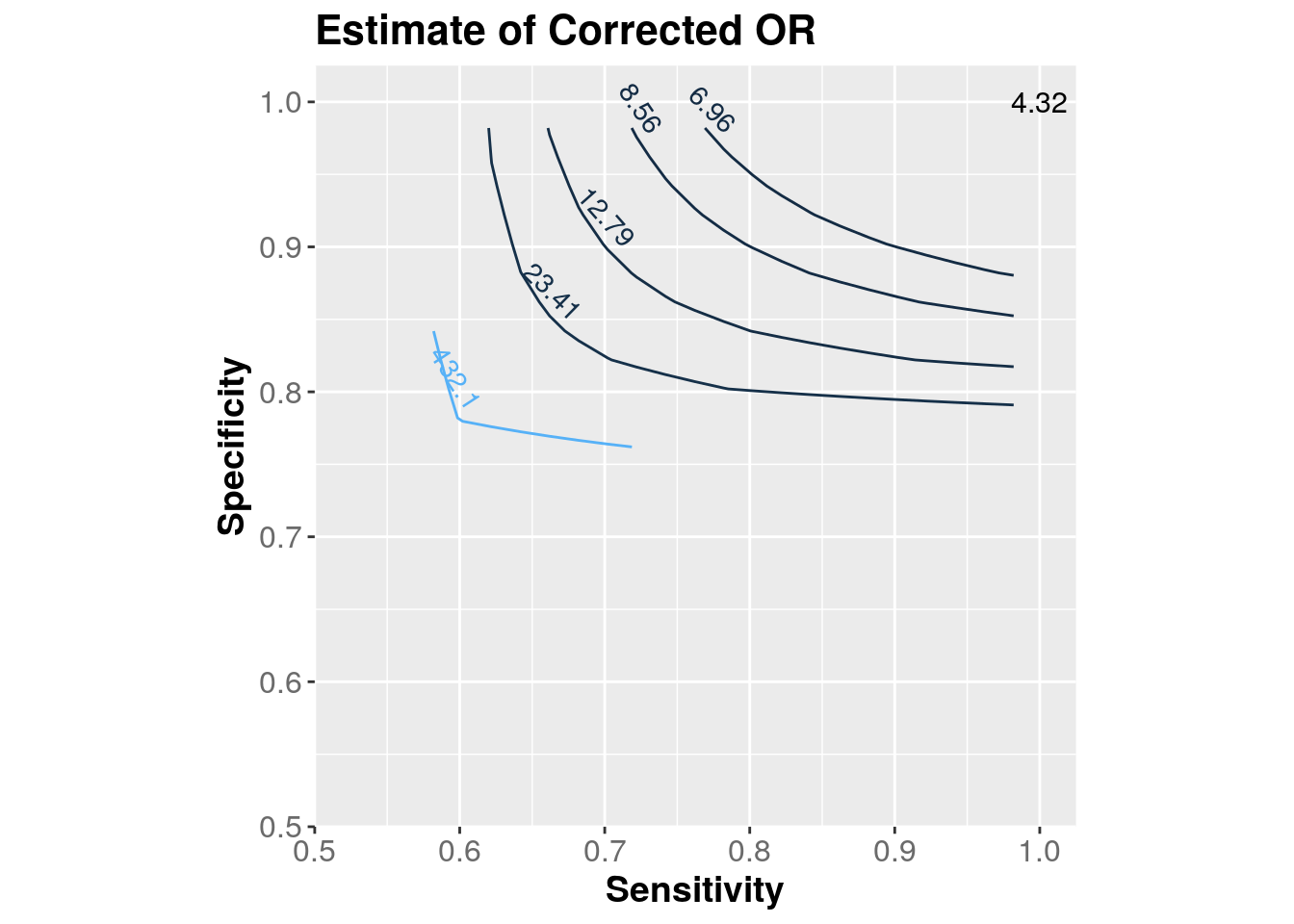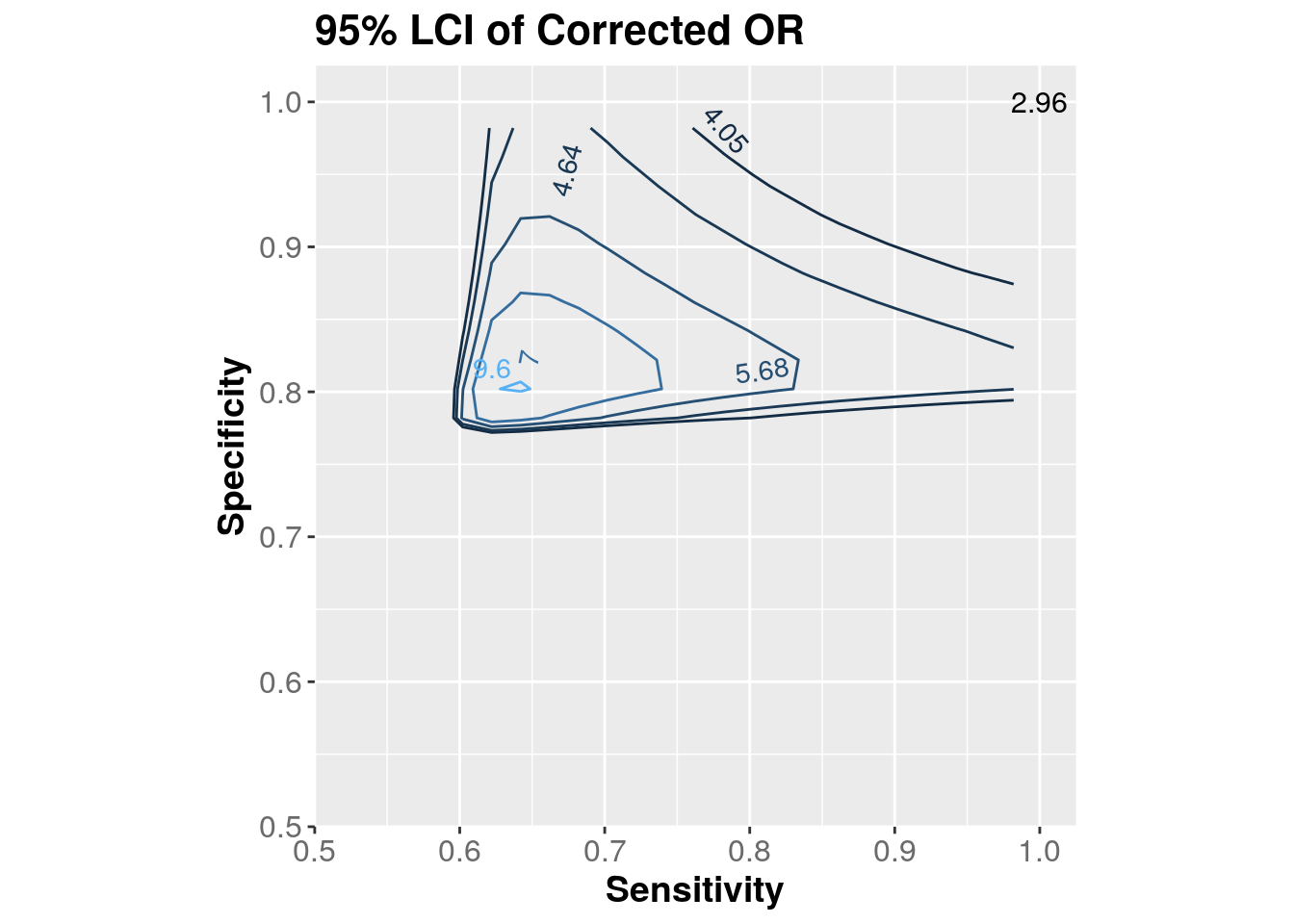# Episensr 0.9.0 now available on CRAN

A small update for my episensr R package is now available on CRAN. The update focus on misclassification.

First, covariate misclassification is now available, via the function misclassification_cov. For example, the paper by Berry et al. looked if misclassification of the confounding variable in vitro fertilization (IVF), a confounder, resulted wrongly on an association between increase folic acid and having twins. IVF increases the risk of twins, and women undergoing IVF might be more likely to take folic acid supplements, i.e. IVF would be a confounder between vitamins and twins. Data on IVF were not available and a proxy for it was used, period of involuntary childlessness. However, it was a poor proxy for IVF, with a sensitivity of 60% and a specificity of 95%. These bias parameters were assumed to be nondifferential. Here’s the code with episensr:

library(episensr)
## Registered S3 methods overwritten by 'ggplot2':
##   method         from
##   [.quosures     rlang
##   c.quosures     rlang
##   print.quosures rlang
misclassification_cov(array(c(1319, 38054, 5641, 405546, 565, 3583,
781, 21958, 754, 34471, 4860, 383588),
dimnames = list(c("Twins+", "Twins-"),
c("Folic acid+", "Folic acid-"),
c("Total", "IVF+", "IVF-")),
dim = c(2, 2, 3)),
bias_parms = c(.6, .6, .95, .95))
## --Observed data--
##          Outcome: Twins+
##        Comparing: Folic acid+ vs. Folic acid-
##
## , , Total
##
##        Folic acid+ Folic acid-
## Twins+        1319        5641
## Twins-       38054      405546
##
## , , IVF+
##
##        Folic acid+ Folic acid-
## Twins+         565         781
## Twins-        3583       21958
##
## , , IVF-
##
##        Folic acid+ Folic acid-
## Twins+         754        4860
## Twins-       34471      383588
##
##
##                                      2.5%    97.5%
## Observed Relative Risk: 2.441910 2.301898 2.590437
##    Observed Odds Ratio: 2.491888 2.344757 2.648251
## ---
##                                                       Observed Corrected
##                       SMR RR adjusted for confounder: 2.261738  1.000235
##    RR due to confounding by misclassified confounder: 1.079661  2.441337
##           Mantel-Haenszel RR adjusted for confounder: 2.228816  1.000187
## MH RR due to confounding by misclassified confounder: 1.095608  2.441452
##                       SMR OR adjusted for confounder: 2.337898  1.000304
##    OR due to confounding by misclassified confounder: 1.065867  2.491131
##           Mantel-Haenszel OR adjusted for confounder: 2.290469  1.000215
## MH OR due to confounding by misclassified confounder: 1.087938  2.491351

While the non-adjusted analysis showed that women taking folic acid were 2.44 times more likely to have twins, after correcting for the misclassification of IVF, risk ratio is now null (= 1.0).

Second, confidence intervals for corrected association due to exposure misclassification are now available. While previously you could already bootstrapped the estimates, you can also now get the confidence intervals from the variance of the corrected odds ratio estimator, as in Greenland et al. and Chu et al.. Using the example in Chu et al. of a case-control study of cigarette smoking and invasive pneumococcal disease, the unadjusted odds ratio is 4.32, with a 95% confidence interval of 2.96 to 6.31. Let’s say the sensitivity of self-reported smoking is 94% and specificity is 97%, for both the case and control groups:

misclassification(matrix(c(126, 92, 71, 224),
dimnames = list(c("Case", "Control"),
c("Smoking +", "Smoking - ")),
nrow = 2, byrow = TRUE),
type = "exposure",
bias_parms = c(0.94, 0.94, 0.97, 0.97))
## --Observed data--
##          Outcome: Case
##        Comparing: Smoking + vs. Smoking -
##
##         Smoking + Smoking -
## Case          126         92
## Control        71        224
##
##                                      2.5%    97.5%
## Observed Relative Risk: 2.196866 1.796016 2.687181
##    Observed Odds Ratio: 4.320882 2.958402 6.310846
## ---
##                                                              2.5%    97.5%
## Misclassification Bias Corrected Relative Risk: 2.377254
##    Misclassification Bias Corrected Odds Ratio: 5.024508 3.282534 7.690912

The corrected odds ratio is now 5.02, with a widened 95% confidence interval (3.28 to 7.69). Note the bias despite the large sensitivity and specificity.

You can even reproduce the contour plots in Chu et al. paper!

dat <- expand.grid(Se = seq(0.582, 1, 0.02),
Sp = seq(0.762, 1, 0.02))

dat$OR_c <- apply(dat, 1, function(x) misclassification(matrix(c(126, 92, 71, 224), nrow = 2, byrow = TRUE), type = "exposure", bias_parms = c(x, x, x, x))$adj.measures[2, 1])
dat$OR_c <- round(dat$OR_c, 2)

library(ggplot2)
library(directlabels)
p1 <- ggplot(dat, aes(x = Se, y = Sp, z = OR_c)) +
geom_contour(aes(colour = ..level..), breaks = c(4.32, 6.96, 8.56, 12.79, 23.41, 432.1)) +
annotate("text", x = 1, y = 1, label = "4.32", size = 4) +
scale_fill_gradient(limits = range(dat$OR_c), high = 'red', low = 'green') + scale_x_continuous(breaks = seq(0.5, 1, .1), expand = c(0,0)) + scale_y_continuous(breaks = seq(0.5, 1, .1), expand = c(0,0)) + coord_fixed(ylim = c(0.5, 1.025), xlim = c(0.5, 1.025)) + scale_colour_gradient(guide = 'none') + theme(plot.title = element_text(face = "bold", size = 16), axis.title = element_text(face="bold", size = 14), axis.text.y = element_text(colour="grey42", size = 12), axis.text.x = element_text(colour="grey42", size = 12), legend.position = "none") + xlab("Sensitivity") + ylab("Specificity") + ggtitle("Estimate of Corrected OR") direct.label(p1, list("far.from.others.borders", "calc.boxes", "enlarge.box", hjust=1, vjust=-.5, box.color = NA, cex = .9, fill = "transparent", "draw.rects"))Figure 1: Contour plot of point estimate of corrected odds ratio (OR) dat$ORc_lci <- apply(dat, 1,
function(x) misclassification(matrix(c(126, 92, 71, 224),
nrow = 2, byrow = TRUE),
type = "exposure",
bias_parms = c(x, x, x, x))$adj.measures[2, 2]) dat$ORc_lci <- round(dat$ORc_lci, 2) p3 <- ggplot(dat, aes(x = Se, y = Sp, z = ORc_lci)) + geom_contour(aes(colour = ..level..), breaks = c(4.05, 4.64, 5.68, 7.00, 9.60)) + annotate("text", x = 1, y = 1, label = "2.96", size = 4) + scale_fill_gradient(limits = range(dat$ORc_lci), high = 'red', low = 'green') +
scale_x_continuous(breaks = seq(0.5, 1, .1), expand = c(0,0)) +
scale_y_continuous(breaks = seq(0.5, 1, .1), expand = c(0,0)) +
coord_fixed(ylim = c(0.5, 1.025), xlim = c(0.5, 1.025)) +
theme(plot.title = element_text(face = "bold", size = 16),
axis.title = element_text(face="bold", size = 14),
axis.text.y = element_text(colour="grey42", size = 12),
axis.text.x = element_text(colour="grey42", size = 12),
legend.position = "none") +
xlab("Sensitivity") +
ylab("Specificity") +
ggtitle("95% LCI of Corrected OR")
direct.label(p3, list("far.from.others.borders", "calc.boxes", "enlarge.box",
hjust=1, vjust=-.5, box.color = NA, cex = .9,
fill = "transparent", "draw.rects"))Figure 2: Contour plot of 95% lower confidence limit of corrected OR